GET THE APP

Numerical Simulation of Influence of Pores Sizes on Water Migration Dynamic in Concrete Building Walls

# Journal of Civil and Environmental Engineering

ISSN: 2165-784X

Open Access
Submit Manuscript arrow_forward arrow_forwardResearch Article - (2020) Volume 10, Issue 6

# Numerical Simulation of Influence of Pores Sizes on Water Migration Dynamic in Concrete Building Walls

Nde NM1*, Fokwa D1, Mbessa M2, Tamo TT2 and Pettang C3
*Correspondence: Nde NM, Assistant Professor, Department of Civil Engineering, University of Douala, Douala, Cameroon, Tel: + 237695966339, Email:
1Department of Civil Engineering, University of Douala, Douala, Cameroon
2Department of Civil Engineering, University of Yaounde, Yaounde, Cameroon
3Department of Civil Engineering, University of Bamenda, Bamenda, Cameroon

## Abstract

The sometimes extreme hydro-climatic stresses that buildings undergo can lead to significant deterioration which can lead to their collapse. The concern to realize durable works and ensuring a comfortable framework for the life of the occupants leads to seek effective solutions, as well for the new construction as for the renovation of old construction, answering the sempiternal problem of harmful action of water on buildings materials. This paper proposes a numerical simulation of moisture migration in concrete building walls, the aim being to highlight the influence of pore size on the kinetics of moisture migration, and its gradient in the wall. A mathematical model taking into account the mechanisms of moisture migration due to liquid moisture gradient and by vapor diffusion is proposed; the discrete formulation of the equation by the numerical scheme of Crank Nicolson is then carried out, and results from computer modeling using Matlab software version 7.10.0.499 (R2010a), show that pore size is a key parameter that influences the dynamics of moisture migration in the wall. Indeed, this parameter qualitatively and quantitatively influences the kinetics of moisture migration, as well as it gradient in the concrete wall.

#### Keywords

Mathematical modelling • Migration • Moisture • Numerical simulation • Pore size

#### Material and Methods

To study the water migration in based of buildings, a mathematical model is proposed and then the numerical simulations are done with Matlab software. The method is based on finite differences and consists in defining an optimal geometry, space and time discretization, initial and boundary conditions.

Mathematical modelling

The model is based on the Philip and De Vries equations; moisture moves in the liquid and vapor state, with the moisture potential as the common potential. In this approach, the scale is assumed to be macroscopic, where the parous medium is considered to be an equivalent fictitious continuous medium; transfers are unsteady and one-dimensional; the different states (liquid and vapor) are in thermal equilibrium at any point in the parous medium; the capillary pores are assumed to be parallel and cylindrical. The liquid and vapor moisture flows are given according to Philip and De Vries  respectively by Equation (1) and Equation (2) while neglecting the gravity flow: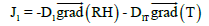(1)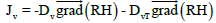(2)

J1 and JV represent the liquid and vapor moisture flow respectively, D1 and DV respectively the liquid and vapor moisture diffusion coefficients. D1T and DVT respectively the liquid and vapor moisture diffusion coefficients due to temperature gradient. T the temperature and RH moisture content (relative humidity).

At the liquid-gas thermodynamic equilibrium, Thomson’s equation  gives the relationship between relative humidity, temperature and pore radius as presented in Equation (3), and transformed to get Equation (4):

PV/ P0V= RH = esp [ (-2×Ts × γw cosβ) / (r×R×T) (3)

T = (-2×Ts ×λw ×cosβ) / (r×R×lnRH) (4)

PV and P0V are respectively the vapor pressure and the saturated vapor pressure; Ts the surface tension; YW the molar volume of water; r the pore radius; R the perfect gas constant and β the water contact angle.

A coupling of Equation (1), Equation (2), and Equation (4) lead to Equation (5):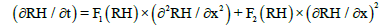(5)

With F1 (RH) and F2 (RH) the moisture migration coefficients, function of the moisture RH and given by the Equation (6) and Equation (7):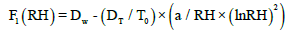(6)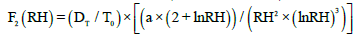(7)

Where a is a coefficient defined to simplify writing. DW the moisture diffusion coefficients due to water content gradient. And DT the moisture diffusion coefficients due to temperature gradient .

a = (-2×Ts × γw ×cosβ) / (r×R) (8)

Dw = D1 + Dv (9)

DT = DIT + DVT (10)

Linearization

The computational of the homogeneous and nonlinear Equation (5) by the finite difference method requires to linearize it. An approximation is therefore made on the coefficients and, they are discrete and supposed known at time j and abscissa i. we then obtain Equation (11) and Equation (12):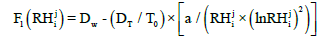(11)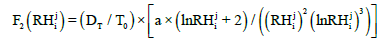(12)

Geometry and discretization

The diagram in Figure 1 illustrates the spatial and temporal discretization of the studied geometry.

The progressive finite differences method is used to discretize moisture flow, given by Equation (13); and the centered finite differences method discretize the moisture gradient, given by Equation (14)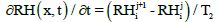(13)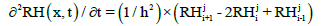(14)

With T2 and h respectively the temporal and spatial discretization rates, defined by Equations (15) and Equation (16):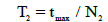(15)

h = e / N1 (16)

e is the wall thickness, tmax the duration of moisture migration, N1 and N2 respectively the number of space and time steps.

Discrete formulation of the equation by Crank Nicolson scheme

For the discrete formulation of Equation (5), the second coefficient F2 (θ) is excluded because its influence on the migration dynamics is negligible. Thus, the discrete scheme of Crank Nicolson, of Equation (17) leads to the algebraic equation system, Equation (18):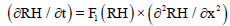(17)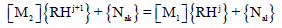(18)

[M1] and [M2] are the material characteristics matrix. The matrix of boundaries conditions are given at time j and j+1 respectively by {Nal} and {Nak}

Initial and boundaries conditions, calculation parameters

The initial conditions and the boundaries conditions in moisture, applied to the geometry are defined as follows:

For x = 0, RH (0, t) = RH0 (t) ∀t ≥ 0 (19)

For x = ∞, RH (∞, t) = RH0 (t) ∀t ≥ 0 (20)

For t = 0, RH (x,0) = RHi t = (0) ∀x (21)

The numerical values of the parameters used in this simulation come from Bordachev’s work on moisture calculation analysis and injection methods in brick masonry walls, where he shows that the revetment based on a material with high porosity or its injection into the wall basement, considerably limits the moisture migration in the wall . Furthermore, some numerical values of parameters were taken from Kiwan’s work, on the reliability of energy performance in buildings . Simulations are performed using Matlab software (version 7.10.0.499 (R2010a)), varying the pore size.

#### Conclusion

This paper presents a numerical simulation of moisture migration in concrete building walls, the influence of pore size on the dynamic of moisture migration has been highlighted, and it appears a low diffusivity in the center of the wall, this independently of pores sizes. Furthermore, a greater migration dynamic when the pores sizes decrease, means a greater kinetics of moisture migration and lower moisture gradient in the walls at the hygrometric equilibrium, for a decreasing pore size. These results find their applications in the choice of building materials their quality, their manufacture and their use for a better durability.

#### Journal Highlights

###### Citations: 1255

Journal of Civil and Environmental Engineering received 1255 citations as per google scholar report